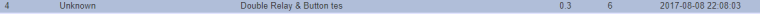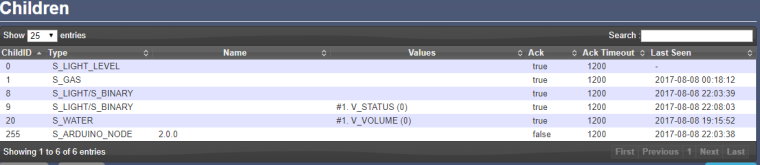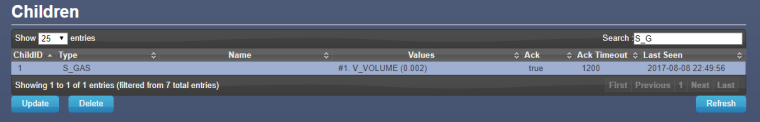# Gateway update?

• Hi,

Here's my debug from Ethernet (Arduino Uno + shield) gateway:

``````0;255;3;0;9;Starting gateway (RNNGA-, 2.0.0)
0;255;3;0;9;TSM:INIT
0;255;3;0;9;TSM:RADIO:OK
0;255;3;0;9;TSM:GW MODE
0;255;3;0;9;TSM:READY
IP: 10.0.0.104
0;255;3;0;9;No registration required
0;255;3;0;9;Init complete, id=0, parent=0, distance=0, registration=1
0;255;3;0;9;TSP:MSG:READ 21-21-0 s=1,c=1,t=0,pt=7,l=5,sg=0:26.0
0;255;3;0;9;TSP:MSG:READ 21-21-0 s=1,c=1,t=0,pt=7,l=5,sg=0:25.9
0;255;3;0;9;TSP:MSG:READ 8-8-0 s=0,c=1,t=37,pt=7,l=5,sg=0:50.87
0;255;3;0;9;TSP:MSG:READ 8-8-0 s=2,c=1,t=0,pt=7,l=5,sg=0:22.0
0;255;3;0;9;TSP:MSG:READ 8-8-0 s=0,c=1,t=37,pt=7,l=5,sg=0:61.50
0;255;3;0;9;TSP:MSG:READ 8-8-0 s=2,c=1,t=0,pt=7,l=5,sg=0:21.9
0;255;3;0;9;TSP:MSG:READ 8-8-0 s=1,c=1,t=1,pt=7,l=5,sg=0:40.7
0;255;3;0;9;TSP:MSG:READ 8-8-0 s=0,c=1,t=37,pt=7,l=5,sg=0:51.34
0;255;3;0;9;TSP:MSG:READ 21-21-0 s=1,c=1,t=0,pt=7,l=5,sg=0:26.0
0;255;3;0;9;TSP:MSG:READ 3-3-0 s=13,c=1,t=0,pt=7,l=5,sg=0:27.7
0;255;3;0;9;TSP:MSG:READ 21-21-0 s=1,c=1,t=0,pt=7,l=5,sg=0:25.9
0;255;3;0;9;TSP:MSG:READ 8-8-0 s=0,c=1,t=37,pt=7,l=5,sg=0:37.04
0;255;3;0;9;TSP:MSG:READ 21-21-0 s=1,c=1,t=0,pt=7,l=5,sg=0:26.0
0;255;3;0;9;TSP:MSG:READ 21-21-0 s=1,c=1,t=0,pt=7,l=5,sg=0:25.9
0;255;3;0;9;TSP:MSG:READ 21-21-0 s=1,c=1,t=0,pt=7,l=5,sg=0:26.0
0;255;3;0;9;TSP:MSG:READ 8-8-0 s=2,c=1,t=0,pt=7,l=5,sg=0:22.0

``````

My nodes are sending data.

Want to add new node and here's the problem:

``````0 MCO:BGN:INIT NODE,CP=RNNNA--,VER=2.1.0
3 TSM:INIT
6 TSF:WUR:MS=0
13 !TSM:INIT:TSP FAIL
15 TSM:FAIL:CNT=1
16 TSM:FAIL:PDT
10019 TSM:FAIL:RE-INIT
10021 TSM:INIT
10028 !TSM:INIT:TSP FAIL
10031 TSM:FAIL:CNT=2
10032 TSM:FAIL:PDT
20035 TSM:FAIL:RE-INIT
20037 TSM:INIT
20043 !TSM:INIT:TSP FAIL
``````

It seems that I have newer version on node (2.1.0) than gateway (2.0.0).

How to fix that?
Should I update gateway - how?
If yes, should I update also all remaning nodes - how?

• @1kohm to update the nodes, re-upload the original sketch to the nodes.

Mixing nodes within the same major version (2.x can coexist) should be OK , so you don't need to update everything.

TSM:INIT:TSP FAIL means that the Arduino is unable to communicate with its radio. Double-check the wiring and if the wiring is correct, try another radio module to determine if the current module is broken.

• Hi,

radio was the issue (placed oposite.., by bad)

Now, I've uploaded node ver.2.0.0.

``````Starting sensor (RNNNA-, 2.0.0)
TSM:INIT
TSM:RADIO:OK
TSP:ASSIGNID:OK (ID=4)
TSM:FPAR
TSP:MSG:SEND 4-4-255-255 s=255,c=3,t=7,pt=0,l=0,sg=0,ft=0,st=bc:
TSP:MSG:READ 0-0-4 s=255,c=3,t=8,pt=1,l=1,sg=0:0
TSP:MSG:FPAR RES (ID=0, dist=0)
TSP:MSG:PAR OK (ID=0, dist=1)
TSM:FPAR:OK
TSM:ID
TSM:CHKID:OK (ID=4)
TSM:UPL
TSP:PING:SEND (dest=0)
TSP:MSG:SEND 4-4-0-0 s=255,c=3,t=24,pt=1,l=1,sg=0,ft=0,st=ok:1
TSP:MSG:READ 0-0-4 s=255,c=3,t=25,pt=1,l=1,sg=0:1
TSP:MSG:PONG RECV (hops=1)
TSP:CHKUPL:OK
TSM:UPL:OK
TSM:READY
TSP:MSG:SEND 4-4-0-0 s=255,c=3,t=15,pt=6,l=2,sg=0,ft=0,st=ok:0100
TSP:MSG:SEND 4-4-0-0 s=255,c=0,t=17,pt=0,l=5,sg=0,ft=0,st=ok:2.0.0
TSP:MSG:SEND 4-4-0-0 s=255,c=3,t=6,pt=1,l=1,sg=0,ft=0,st=ok:0
TSP:MSG:READ 0-0-4 s=255,c=3,t=15,pt=6,l=2,sg=0:0100
TSP:MSG:READ 0-0-4 s=255,c=3,t=6,pt=0,l=1,sg=0:M
TSP:MSG:SEND 4-4-0-0 s=255,c=3,t=11,pt=0,l=25,sg=0,ft=0,st=ok:Double Relay & Button tes
TSP:MSG:SEND 4-4-0-0 s=255,c=3,t=12,pt=0,l=3,sg=0,ft=0,st=ok:0.3
TSP:MSG:SEND 4-4-0-0 s=8,c=0,t=3,pt=0,l=0,sg=0,ft=0,st=ok:
TSP:MSG:SEND 4-4-0-0 s=9,c=0,t=3,pt=0,l=0,sg=0,ft=0,st=ok:
Request registration...
TSP:MSG:SEND 4-4-0-0 s=255,c=3,t=26,pt=1,l=1,sg=0,ft=0,st=ok:2
TSP:MSG:READ 0-0-4 s=255,c=3,t=27,pt=1,l=1,sg=0:1
Node registration=1
Init complete, id=4, parent=0, distance=1, registration=1
``````

Debug looks perfect..., but cannot see the node in Domoticz i Devices tabI can see the node under Hardware -> MySensorsGateway only:I want to test gas meter IN-Z61.

• @1kohm great work.

For some reason, Domoticz does not show the sensor in the devices list until the sensor has sent its first value. (presentation is not enough, a message needs to be sent). Could that be the case for you?

• Hi,

so my node is sending data every 3 seconds

``````TSP:MSG:SEND 7-7-0-0 s=1,c=1,t=24,pt=5,l=4,sg=0,ft=0,st=ok:2
volume:0.002
TSP:MSG:SEND 7-7-0-0 s=1,c=1,t=35,pt=7,l=5,sg=0,ft=0,st=ok:0.002
``````Domoticz see the updated vaule, but not in Devices• Want to present the device as S_GAS

``````void presentation()
{
// Send the sketch version information to the gateway and Controller
sendSketchInfo("Gas Meter", "1.1");

// Register this device as Waterflow sensor
present(CHILD_ID, S_GAS);
}
``````

Sketch I use:

``````/**
* The MySensors Arduino library handles the wireless radio link and protocol
* between your home built sensors/actuators and HA controller of choice.
* The sensors forms a self healing radio network with optional repeaters. Each
* repeater and gateway builds a routing tables in EEPROM which keeps track of the
* network topology allowing messages to be routed to nodes.
*
* Created by Henrik Ekblad <henrik.ekblad@mysensors.org>
* Copyright (C) 2013-2015 Sensnology AB
* Full contributor list: https://github.com/mysensors/Arduino/graphs/contributors
*
* Documentation: http://www.mysensors.org
* Support Forum: http://forum.mysensors.org
*
* This program is free software; you can redistribute it and/or
* modify it under the terms of the GNU General Public License
* version 2 as published by the Free Software Foundation.
*
*******************************
*
* REVISION HISTORY
* Version 1.0 - Henrik Ekblad
* Version 1.1 - GizMoCuz
*
* DESCRIPTION
* Use this sensor to measure volume and flow of your house watermeter.
* You need to set the correct pulsefactor of your meter (pulses per m3).
* The sensor starts by fetching current volume reading from gateway (VAR 1).
* Reports both volume and flow back to gateway.
*
* Unfortunately millis() won't increment when the Arduino is in
* sleepmode. So we cannot make this sensor sleep if we also want
* to calculate/report flow.
* http://www.mysensors.org/build/pulse_water
*/

// Enable debug prints to serial monitor
#define MY_DEBUG

// Enable and select radio type attached
#define MY_RADIO_NRF24
//#define MY_RADIO_NRF5_ESB
//#define MY_RADIO_RFM69
//#define MY_RADIO_RFM95

#include <MySensors.h>

#define DIGITAL_INPUT_SENSOR 7                  // The digital input you attached your sensor.  (Only 2 and 3 generates interrupt!)

#define PULSE_FACTOR 1000                       // Nummber of blinks per m3 of your meter (One rotation/liter)

#define SLEEP_MODE false                        // flowvalue can only be reported when sleep mode is false.

#define MAX_FLOW 40                             // Max flow (l/min) value to report. This filters outliers.

#define CHILD_ID 1                              // Id of the sensor child

unsigned long SEND_FREQUENCY =
3000;           // Minimum time between send (in milliseconds). We don't want to spam the gateway.

MyMessage flowMsg(CHILD_ID,V_FLOW);
MyMessage volumeMsg(CHILD_ID,V_VOLUME);
MyMessage lastCounterMsg(CHILD_ID,V_VAR1);

double ppl = ((double)PULSE_FACTOR)/1000;        // Pulses per liter

volatile unsigned long pulseCount = 0;
volatile unsigned long lastBlink = 0;
volatile double flow = 0;
bool pcReceived = false;
unsigned long oldPulseCount = 0;
unsigned long newBlink = 0;
double oldflow = 0;
double volume =0;
double oldvolume =0;
unsigned long lastSend =0;
unsigned long lastPulse =0;

void setup()
{
// initialize our digital pins internal pullup resistor so one pulse switches from high to low (less distortion)
pinMode(DIGITAL_INPUT_SENSOR, INPUT_PULLUP);

pulseCount = oldPulseCount = 0;

// Fetch last known pulse count value from gw
request(CHILD_ID, V_VAR1);

lastSend = lastPulse = millis();

attachInterrupt(digitalPinToInterrupt(DIGITAL_INPUT_SENSOR), onPulse, FALLING);
}

void presentation()
{
// Send the sketch version information to the gateway and Controller
sendSketchInfo("Gas Meter", "1.1");

// Register this device as Waterflow sensor
present(CHILD_ID, S_GAS);
}

void loop()
{
unsigned long currentTime = millis();

// Only send values at a maximum frequency or woken up from sleep
if (SLEEP_MODE || (currentTime - lastSend > SEND_FREQUENCY)) {
lastSend=currentTime;

if (!pcReceived) {
//Last Pulsecount not yet received from controller, request it again
request(CHILD_ID, V_VAR1);
return;
}

if (!SLEEP_MODE && flow != oldflow) {
oldflow = flow;

Serial.print("l/min:");
Serial.println(flow);

// Check that we dont get unresonable large flow value.
// could hapen when long wraps or false interrupt triggered
if (flow<((unsigned long)MAX_FLOW)) {
send(flowMsg.set(flow, 2));                   // Send flow value to gw
}
}

// No Pulse count received in 2min
if(currentTime - lastPulse > 120000) {
flow = 0;
}

// Pulse count has changed
if ((pulseCount != oldPulseCount)||(!SLEEP_MODE)) {
oldPulseCount = pulseCount;

Serial.print("pulsecount:");
Serial.println(pulseCount);

send(lastCounterMsg.set(pulseCount));                  // Send  pulsecount value to gw in VAR1

double volume = ((double)pulseCount/((double)PULSE_FACTOR));
if ((volume != oldvolume)||(!SLEEP_MODE)) {
oldvolume = volume;

Serial.print("volume:");
Serial.println(volume, 3);

send(volumeMsg.set(volume, 3));               // Send volume value to gw
}
}
}
if (SLEEP_MODE) {
sleep(SEND_FREQUENCY);
}
}

void receive(const MyMessage &message)
{
if (message.type==V_VAR1) {
unsigned long gwPulseCount=message.getULong();
pulseCount += gwPulseCount;
flow=oldflow=0;
Serial.print("Received last pulse count from gw:");
Serial.println(pulseCount);
pcReceived = true;
}
}

void onPulse()
{
if (!SLEEP_MODE) {
unsigned long newBlink = micros();
unsigned long interval = newBlink-lastBlink;

if (interval!=0) {
lastPulse = millis();
if (interval<500000L) {
// Sometimes we get interrupt on RISING,  500000 = 0.5sek debounce ( max 120 l/min)
return;
}
flow = (60000000.0 /interval) / ppl;
}
lastBlink = newBlink;
}
pulseCount++;
}
``````

• topic to close.
I've neede to allow new hardware to add in Domoticz.